Courses

# Doc: Electrolytic Cells and Electrolysis Class 12 Notes | EduRev

## Chemistry Class 12

Created by: Mohit Rajpoot

## Class 12 : Doc: Electrolytic Cells and Electrolysis Class 12 Notes | EduRev

The document Doc: Electrolytic Cells and Electrolysis Class 12 Notes | EduRev is a part of the Class 12 Course Chemistry Class 12.
All you need of Class 12 at this link: Class 12

Electrolysis

The decomposition of electrolyte solution by passage of electric current, resulting into deposition of metals or liberation of gases at electrodes is known as electrolysis.

Electrolytic cell

This cell converts electrical energy into chemical energy. The entire assembly except that of the external battery is known as the electrolytic cell.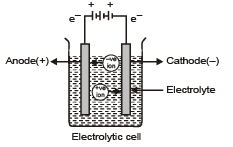Electrodes

The metal strip at which positive current enters is called anode; anode is positively charged in electrolytic cell. On the other hand, the electrode at which current leaves is called cathode. Cathodes are negatively charged.

 Anode Positive Loss of electron or oxidation takes place Positive Current enters Cathode Negative Gain of electron or reduction takes place Current leaves

Electrolysis of molten sodium chloride

NaCl(molten) → Na  + Cl-

Reactions at anode (oxidation) ; cathode (reduction)

2Cl- → Cl2(g)  + 2e- ; 2Na  + 2e- → 2Na(l)

There are two types of electrodes used in the electrolytic cell, namely attackable and non-attackable. The attackable electrodes participitate in the electrode reaction. They are made up of reactive metals like Zn, Cu, Ag etc. In such electrodes, atom of the metal gets oxidised into the corresponding cation, which is passed into the solution. Thus, such anodes get dissolved and their mass decreases. On the other hand, non-attackable electrodes do not participate in the electrodes do not participate in the electrode reaction as they made up of unreactive elements like Pt, graphite etc. Such electrodes do not dissolve and their mass remain same.

(i) First law of electrolysis;

Amount of substance deposited or liberated at an electrode is directly proportional to amount of charge passed (utilized) through the solution.

w ∝ Q

W = weight liberated, Q = charge in coulomb

w = ZQ

Z = electrochemical equivalent

when Q = 1 coulomb, then w = Z

Thus, weight deposited by 1 coulomb charge is called electrochemial equivalent.

Let 1 ampere current is passed till 't' seconds.

Then, Q = It w = ZIt

1 Faraday = 96500 coulomb = Charge of one mole electrons

One faraday is the charge required to liberate or deposit one gm equivalent of a substance at corresponding electrode.

Let 'E' is equivalent weight then 'E' gm will be liberated by 96500 coulomb.

1 Coulomb will liberate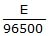gm ; By definition, Z =W =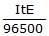When a gas is evolved at an electrode, then above formula changes as,

V =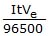where V = volume of liberated gas, Ve = equivalent volume of gas.

Equivalent volume may be defined as:

The volume of gas liberated by 96500 coulomb at STP.

(ii) Second law of electrolysis :

When same amount of charge is passed through different electrolyte solutions connected in series then weight of substances deposited or dissolved at anode or cathode are in ratio of their equivalent weights. i.e. w1/w2 = E1/E2

Qualitative aspects of electrolysis

In the electrolysis process we have discussed above, we have taken molten salt as electrolyte, which contains only one cation and anion. Now, if the electrolyte taken contains more than one cation and anion (for example, aqueous solution of the ionic electrolyte), then the cation and anion that will get discharged depends on the ability of cation to get reduced and the ability of anion to get oxidized.

The ability of an ion to get oxidized or reduced depends upon the size, mass, positive charge, negative charge etc. Thus it is not possible to predict qualitatively that which ion would be discharged first, as one factor might enhance the ability to discharge while the other factor may hamper it. This can only be predicted on the basis of quantitative value assigned based on the cumulative effect of all the factors responsible for an ion's ability of discharge. The value is referred as standard potential, which is determined by keeping the concentration of ion as 1 M, pressure of gas at 1 atm, and the measurement done at 25ºC. For a cation, the standard reduction potential (SRP) values are compared. The cation having higher standard reduction potential value is discharged in preference to cation with lower SRP value provided the ions are at 1M concentration. For an anion, the standard oxidation potential (SOP) values are compared and anion having higher SOP is preferentially discharged, if the concentration is 1 M for each of the ion. The SRP Values at 25º C for some of the reduction half reaction are given in the table below.

 S. NO. Reduction half cell reaction Eo in volts at 250 1. F2 + 2e- →→  2F- + 2.65 2. S2O82- + 2e- → 2SO42- + 2.01 3. Co3+ + e- → Co2+ + 1.82 4. PbO2 + 4H+ + SO42- + 2e- → PbSO4 + 2H2O + 1.65 5. MnO4- + 8H+ + 5e- → Mn2+ + 4H2O + 1.52 6. Au3+ + 3e- → Au + 1.50 7. Cl2 + 2e- → 2Cl- + 1.36 8. Cr2O2-7 + 14 H+ + 6e- → 2Cr3+ + 7H2O + 1.33 9. O2 + 4H+ + 4e- → 2H2O + 1.229 10. Br2 + 2e- → 2Br- + 1.07 11. NO3- + 4H+ + 3e- → NO + 2H2O + 0.96 12. 2Hg2+ + 2e- → Hg22+ + 0.92 13. Cu2+ + I- + e- → CuI + 0.86 14. Ag+ + e- → Ag + 0.799 15. Hg22+ + 2e- → 2Hg + 0.79 16. Fe3+ + e- → Fe2+ + 0.77 17. I2 + 2e- → 2I- + 0.535 18. Cu+ + e- → Cu + 0.53 19. Cu2+ + 2e- → Cu + 0.34 20. Hg2Cl2 + 2e- → 2Hg + 2Cl- + 0.27 21. AgCl + e- → Ag + Cl- + 0.222 22. Cu2+ + e- → Cu+ + 0.15
 23 Sn4+ + 2e- → Sn2+ + 0.13 24 2H+ + 2e- → H2 + 0.00 25 Fe3+ + 3e- → Fe - 0.036 26 Pb2+ + 2e- → Pb - 0.126 27 Sn2+ + 2e- → Sn - 0.14 28 Agl + e- → Ag + I- - 0.151 29 Ni2+ + 2e- → Ni - 0.25 30 Co2+ + 2e- → Co - 0.28 31 Cd2+ + 2e- → Cd - 0.403 32 Cr3+ + e- → Cr2+ - 0.41 33 Fe2+ + 2e- → Fe - 0.44 34 Cr3+ + 3e- → Cr - 0.74 35 Zn2+ + 2e- → Zn - 0.762 36 2H2O + 2e- → H2 + 2OH- - 0.828 37 Mn2+ + 2e- → Mn - 1.18 38 Al3+ + 3e- → Al - 1.66 39 H2 + 2e- → 2H- - 2.25 40 Mg2+ + 2e- → Mg - 2.37 41 Na+ + e- → Na - 2.71 42 Ca2+ + e- → Ca - 2.87 43 Ba2+ + 2e- → Ba - 2.90 44 Cs+ + e- → Cs - 2.92 45 K+ + e- → K - 2.93 46 Li+ + e- → Li - 3.03

When solution of an electrolyte contains more than one type of cations and anions at concentrations different than 1 M, the discharge of an ion does not depend solely on standard potentials but also depends on the concentration of ion in the solution. The value is referred as potential, called as reduction potential for cation and oxidation potential for anion. The relation between reduction potential and standard reduction potential is given by Nernest equation, as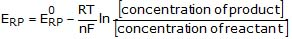Where ERP = Reduction potential of cation and EºRP = Standard reduction potential of cation. Thus, it is possible that a cation (A+ ) with lower standard reduction potential getting descharged in preference to cation (B+) having higher standard reduction potential because their concentration might be such that the reduction potential of A  is higher than that of B+ .
When two metal ions in the solution have identical values of their reduction potentials, the simultaneous deposition of both the metals will occur in the form of an alloy.

Offer running on EduRev: Apply code STAYHOME200 to get INR 200 off on our premium plan EduRev Infinity!

,

,

,

,

,

,

,

,

,

,

,

,

,

,

,

,

,

,

,

,

,

;